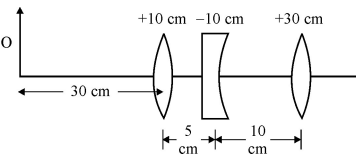# (a) Under what conditions is the phenomenon of total internal reflection of light observed? Obtain the relation between the critical angle of incidence and the refractive index of the medium.(b) Three lenses of focal lengthsandare arranged coaxially as in the figure given below. Find the position of the final image formed by the combination.(a) The conditions for the total internal reflection of light are :

(i) The angle of incidence must be greater than a certain angle (critical angle).

(ii) The light must be trying to travel from optically denser medium to optically lighter medium.

Now, the Relation :

Let us consider the two mediums, mediumand mediumin which the light is travelling fromtomedium. Here medium one is denser than medium 2.

Note that, when light strikes the surface separating the media, some rays of it reflects and some of it refracts.

If we continue increasing the angle of incidence, then the refracted ray becomes parallel to the surface. At this point, the value obtained for the angle of incidence is known as the critical angle.

Now, if we keep increasing the angle of incidence, even further, then we will only obtain reflected ray no refracted ray.

Thus, we will have a total internal reflection.

Now, let's consider the condition, when the angle of incidence is equal to the critical angleSo, By Snell's law, we have(b) We already know the lens formula :-Where,is object distance,is image distance, andis focal length

Now, according to the Question,

The first lens will form an image of the given object

At,____given

So, by using the lens formula, we can calculateNow, the object for the second lens would be the image formed by the first lens.

So, Here,orNow, we consider the third lens, Suppose it a converging lens and has a position focal point.

and we know,

An object, when placed at infinity, would have its image at the focal point.

Hence, At either side, from the third lens, the final image will be formed at a distance of.

## Related Chapters

### Preparation Products

##### Knockout CUET (Physics, Chemistry and Mathematics)

Complete Study Material based on Class 12th Syllabus, 10000+ Question Bank, Unlimited Chapter-Wise and Subject-Wise Mock Tests, Study Improvement Plan.

₹ 7999/- ₹ 4999/-
##### Knockout CUET (Physics, Chemistry and Biology)

Complete Study Material based on Class 12th Syllabus, 10000+ Question Bank, Unlimited Chapter-Wise and Subject-Wise Mock Tests, Study Improvement Plan.

₹ 7999/- ₹ 4999/-
##### Knockout JEE Main (Six Month Subscription)

- AI Coach Study Modules, - Unlimited Mock Tests, - Expert Mentorship, - Study Improvement Plan.

₹ 9999/- ₹ 8499/-
##### Knockout JEE Main (Nine Month Subscription)

- AI Coach Study Modules, - Unlimited Mock Tests, - Expert Mentorship, - Study Improvement Plan.

₹ 13999/- ₹ 12499/-